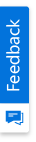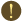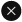We use cookies to give you the best experience on our website. If you continue to browse, then you agree to our privacy policy and cookie policy.Unfortunately, activation email could not send to your email. Please try again.# Essential Studio for HTML5 ASP.NET CoreStacked Line Chart and Graph

## Overview

ASP.NET Core Stacked line chart is chart with Y values stacked over one another in the series order. Shows the relation between individual values to the total sum of the points.## Marker

Marks data points with built-in shapes such as circles, rectangles, ellipses, vertical lines, horizontal lines, diamonds, triangles, and pentagons. In addition to these shapes, use images to make the point more attractive.## Data label

Data labels display information about data points. Add a template to display data labels with HTML elements such as images, DIV, and spans for more informative data labels. You can rotate a data label by its given angle.## Multiple axes

Use multiple axes to plot different data sets that widely vary from one other.## Customization

Customize the look and feel of the stacked line chart using built-in APIs.## Code snippet

```<ejs-chart id="container">
<e-chart-primaryxaxis valueType="Category">
</e-chart-primaryxaxis>
<e-series-collection>
<e-series dataSource="ViewBag.dataSource" name="John" xName="x" width="2" yName="y" type="StackingLine" dashArray="5,1">
<e-series-marker visible="true"></e-series-marker>
</e-series>
<e-series dataSource="ViewBag.dataSource" name="Peter" xName="x" width="2" yName="y1" type="StackingLine" dashArray="5,1">
<e-series-marker visible="true"></e-series-marker>
</e-series>
<e-series dataSource="ViewBag.dataSource" name="Steve" xName="x" width="2" yName="y2" type="StackingLine" dashArray="5,1">
<e-series-marker visible="true"></e-series-marker>
</e-series>
<e-series dataSource="ViewBag.dataSource" name="Charle" xName="x" width="2" yName="y3" type="StackingLine" dashArray="5,1">
<e-series-marker visible="true"></e-series-marker>
</e-series-collection>
</ejs-chart>```
```public class HomeController : Controller
{
public ActionResult Index()
{
List<StackedLineChartData> chartData = new List<StackedLineChartData>
{
new StackedLineChartData { x = "Food" , y = 90, y1 = 40 , y2 = 70, y3 = 120},
new StackedLineChartData { x = "Transport", y = 80, y1 = 90, y2 = 110, y3 = 70 },
new StackedLineChartData { x = "Medical",y = 50, y1 = 80, y2 = 120, y3 = 50 },
new StackedLineChartData { x = "Clothes",y = 70, y1 = 30, y2 = 60, y3 = 180 },
new StackedLineChartData { x = "Personal Care", y = 30, y1 = 80, y2 = 80, y3 = 30 },
new StackedLineChartData { x = "Books", y = 10, y1 = 40, y2 = 30, y3 = 270},
new StackedLineChartData { x = "Fitness",y = 100, y1 = 30, y2 = 70, y3 = 40 },
new StackedLineChartData { x = "Electricity", y = 55, y1 = 95, y2 = 55, y3 = 75},
new StackedLineChartData { x = "Tax", y = 20, y1 = 50, y2 = 40, y3 = 65 },
new StackedLineChartData { x = "Pet Care", y = 40, y1 = 20, y2 = 80, y3 = 95 },
new StackedLineChartData { x = "Education", y = 45, y1 = 15, y2 = 45, y3 = 195 },
new StackedLineChartData { x = "Entertainment", y = 75, y1 = 45, y2 = 65, y3 = 115 }
};
ViewBag.dataSource = chartData;
return View();
}
}
public class StackedLineChartData
{
public string x;
public double y;
public double y1;
public double y2;
public double y3;
}```

## Learning ResourcesStacked Line Chart User Guide

Learn the available options to customize ASP.NET Core stacked line chart.Stacked Line Chart API Reference

Explore the ASP.NET Core stacked line chart APIs.

### 80+ ASP.NET CORE UI CONTROLS

ALL CONTROLS
VIEWER
DOCUMENT PROCESSING LIBRARIES
REPORTSYou are using an outdated version of Internet Explorer that may not display all features of this and other websites. Upgrade to Internet Explorer 8 or newer for a better experience.